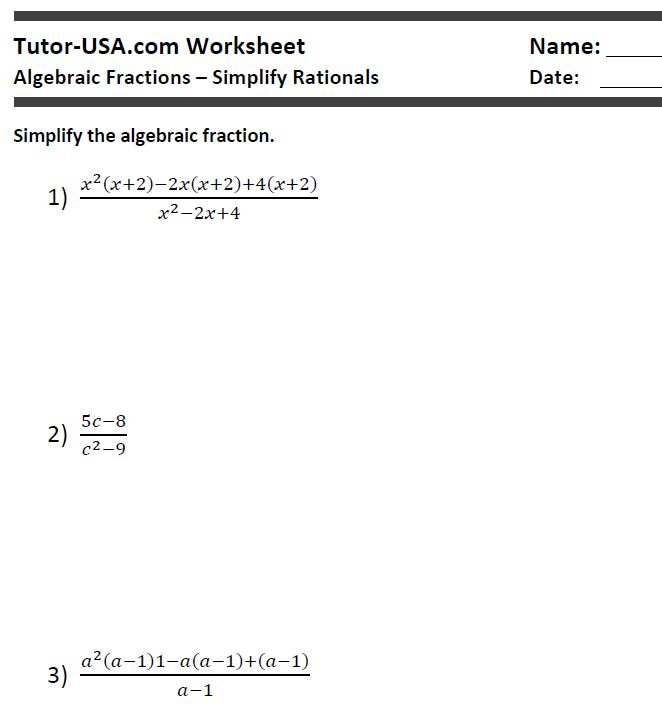# Algebraic Equations - Simplify Rational Expressions

Worksheet (Algebra)

## Algebraic Equations - Simplify Rational Expressions Worksheet

In this free algebra worksheet, students must simplify algebraic expressions by use of the distributive property, combining like terms, cancellation, and other algebra techniques.

## Lesson:

To simplify algebraic fractions or rational expressions.

10

3

Yes

## Samples:

Tutor-USA.com Worksheet
Algebraic Fractions – Simplify Rationals

Simplify the algebraic fraction.

Worksheet Screenshot: